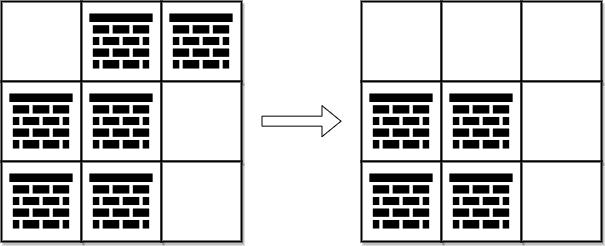2290. Minimum Obstacle Removal to Reach Corner
Hard
743
14

You are given a 0-indexed 2D integer array `grid` of size `m x n`. Each cell has one of two values:

• `0` represents an empty cell,
• `1` represents an obstacle that may be removed.

You can move up, down, left, or right from and to an empty cell.

Return the minimum number of obstacles to remove so you can move from the upper left corner `(0, 0)` to the lower right corner `(m - 1, n - 1)`.

Example 1:```Input: grid = [[0,1,1],[1,1,0],[1,1,0]]
Output: 2
Explanation: We can remove the obstacles at (0, 1) and (0, 2) to create a path from (0, 0) to (2, 2).
It can be shown that we need to remove at least 2 obstacles, so we return 2.
Note that there may be other ways to remove 2 obstacles to create a path.
```

Example 2:```Input: grid = [[0,1,0,0,0],[0,1,0,1,0],[0,0,0,1,0]]
Output: 0
Explanation: We can move from (0, 0) to (2, 4) without removing any obstacles, so we return 0.
```

Constraints:

• `m == grid.length`
• `n == grid[i].length`
• `1 <= m, n <= 105`
• `2 <= m * n <= 105`
• `grid[i][j]` is either `0` or `1`.
• `grid == grid[m - 1][n - 1] == 0`
Accepted
16.8K
Submissions
33.6K
Acceptance Rate
49.9%

Seen this question in a real interview before?
1/4
Yes
No

Discussion (0)

Related Topics